# AP Board 3rd Class Maths Solutions 1st Lesson Let’s Recall

Andhra Pradesh AP Board 3rd Class Maths Solutions 1st Lesson Let’s Recall Textbook Exercise Questions and Answers.

## AP State Syllabus 3rd Class Maths Solutions Chapter 1 Let’s Recall

Textbook Page No. 1

I.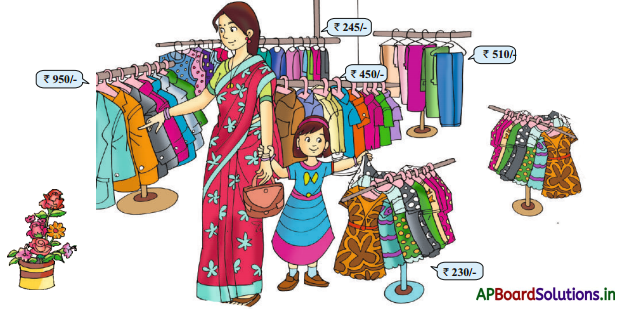Suma and her daughter Manu went for shopping on a Sunday to buy some dresses and vegetables. At first they entered a garment shop and looked at the prices of various dresses.

Question 1.
How many frocks are there ?
16 frocks are there

Question 2.
How many pants are there ?
5 pants are there.

Question 3.
What are the other items you can see in the shop ?
Suits, shirts, long tracks are also in the shop.Question 4.
What is the price of the frock ?
The cost of each frock is ₹230.

Question 5.
Have you ever gone to market ? What did you buy there ?
Yes, I went to market to buy vegetables and fruits.

Textbook Page No. 2

II. Suma paid the amount for frock as shown below.She has ₹100, ₹10 notes only available in her purse. What notes should be given to purchase the following items ?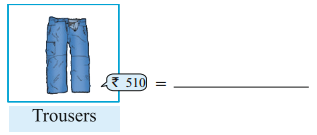Trousers : ₹ 100 + ₹ 100 + ₹ 100 + ₹ 100 + ₹ 100 + ₹ 10 = ₹ 510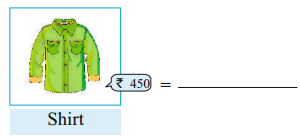Shirt : ₹ 100 + ₹ 100 + ₹ 100 + ₹ 100 + ₹ 10 + ₹ 10 + ₹ 10 + ₹ 10 + ₹ 10
= ₹ 450Do these:

1. Write the following number in words.

a) 4
Four

b) 9
Nine

c) 14
Fourteen

d) 19
Nineteen

e) 28
Twenty eight

f) 46
Forty six

g) 76
Seventy Six

h) 147
One hundred and forty Seven

i) 263
Two hundred and Sixty three

j) 471
Four hundred and Seventy One

k) 683
Six hundred and eighty three

l)750
Seven hundred and fifty

m) 806
Eight hundred and six

n) 975
Nine hundred and Seventy five

2. Write the number of the followings.

a) Eight
8

b) Fifteen
15

c) Nineteen
19

d) Seventy
70e) Eighty six
86

f) Three hundred and sixty five
365

g) Five hundred and seventy nine
579

3. Complete the following table.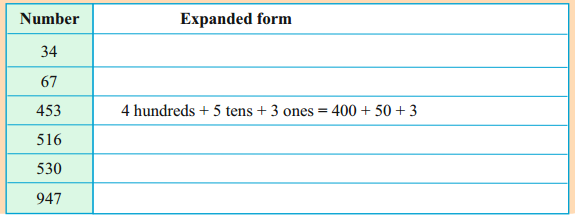Textbook Page No. 3

III. Observe the following base 10 blocks and write the numbers.

Ex: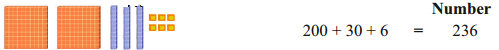Question 1.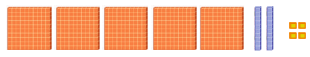_____ + ______ + ______ = _______
500 + 20 + 4 = 524

Question 2.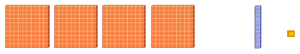_____ + _____ + _____ = ______
400 + 10 + 1 = 411

Question 3.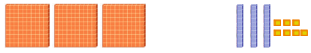_____ + _____ + _____ = ______
300 + 30 + 7 = 337

Textbook Page No. 4

Exercise – 1

1.
a) Identify and colour the boxes with the number that have these same digit in ones and tens place.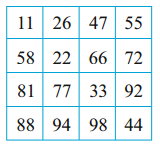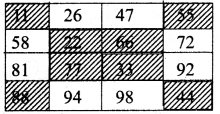b) Colour the boxes with the number that have “3” in tens place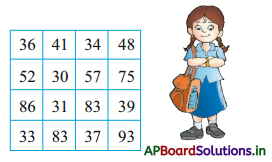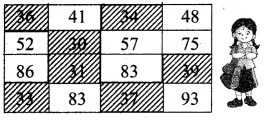2. Write the face value and place values of underlined digits in the following numbers.

88:
Face value = 8
Place value = 80

61:
Face value = 1
Place value = 1

560:
Face value = 5
Place value = 500

725 :
Face value = 2
Place value = 20

3. Observe the prices: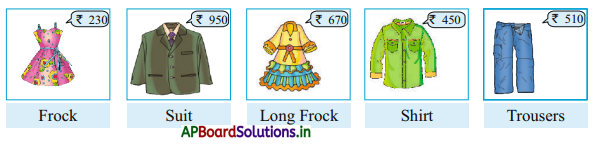a) What is the price of a long frock ?
₹ 670

b) Which one is of the lowest price ?
Frock (₹ 230)c) Which one is of the highest price ?
Suit

d) Which one is cheaper between shirt and trousers ?
Shirt

e) Arrange the above items from lowest to highest price.
Frock, Shirt, Trousers, Long frock, Suit

Textbook Page No. 5

4. Compare the numbers with suitable symbols (<, >, =)

a) 8 < 10

b) 10 < 12

c) 78 > 67

d) 128 < 256e) 8690 < 639

f) 900 = 0900

5. Write the numbers in the ascending order.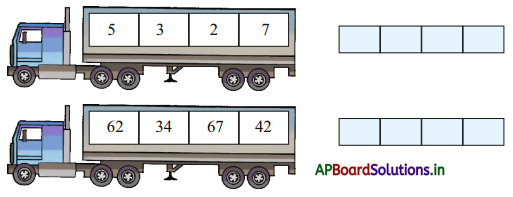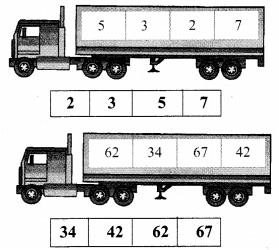6. Write the numbers in the descending order.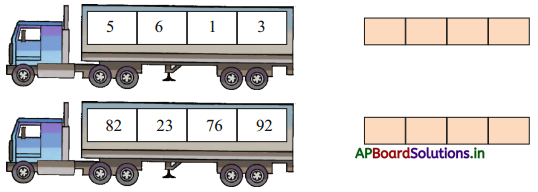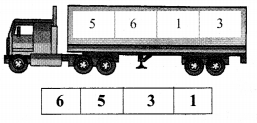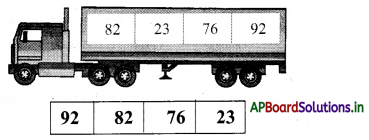7. Write the given numbers in ascending and descending order.

a) 384, 648, 438, 583, 689
Ascending order:
384, 438, 583, 648, 689
Descending order:
689, 648, 583, 438, 384

b) 684,568,796,769,830
Ascending order:
568, 684, 769, 796, 830
Descending order:
830, 796, 769, 684, 568

Textbook Page No. 6

8. Write the 3 digit number which has 7 in hundreds place, 5 in tens place and 6 is one place ?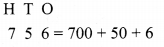Textbook Page No. 7

Do these:

1. Do the followings.

a)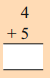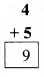b)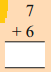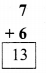c)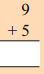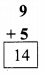d)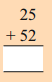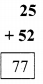e)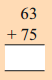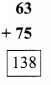f)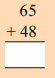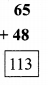g)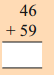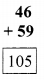h)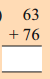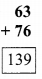i)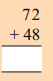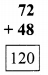j)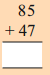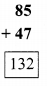k)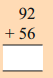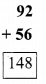l)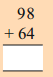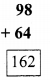2. If they buy the vegetables in the following ways, how much money do they have to pay? Solve the following questions.

a) Find the total price of 1 kg. chillies and 1 kg ridge gourds.
Price of 1 kg chilles = ₹ 20
Price of 1 kg ridge gourds (+) = ₹ 54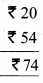The total price of two vegetables = ₹ 74

b) Find the total price of one piece of pumpkin and a dozen lemons.
Price of one piece of pumpkin = ₹ 65
Price of one dozen lemons (+) = ₹ 26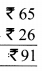The total price of two vegetables = ₹ 91

c) Find the total price of 1 kg. of tomatoes and a snake gourd.
Price of 1 Kg tomatoes = ₹ 18
Price of each snake ground (+) = ₹ 18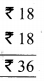The total price of two vegetables = ₹ 36

3. Fill in the boxes with numbers to make the sum equal to 100.

60 + ___ = 100
40

10 + ___ = 100
90

70 + ____ = 100
30

40 + ____ = 100
6050 + ____ = 100
50

80 + ___ = 100
20

30 + ____ = 100
70

90 + ___ = 100
10

20 + ___ = 100
80

Textbook Page No. 8

Do these :

Question 1.
Suma took 1 kg Brinjal and give ₹ 40, how much money will she get back?
Amount paid by Suma = ₹ 40
Price of 1 Kg Brinjal = ₹ 30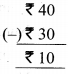Money get back by Suma = ₹ 10

Question 2.
What is the difference between the cost of 1 kg ladies fingers and 1 kg of brinjals?
A. Cost of 1 Kg of ladies fingers = ₹ 33
Cost of 1 Kg of brinjals = ₹ 30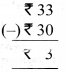Difference between this costs = ₹ 3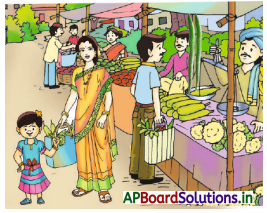If, Suma buy a snake gourd and gives 50 rupee note. How much money will she get back?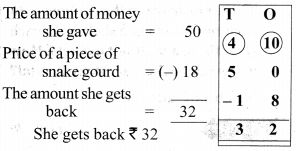Do these :

Question 1.
Subtract the following.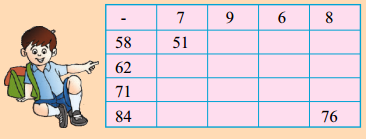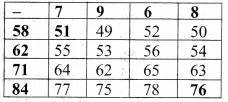Question 2.
Can Suma buy a pumpkin with ₹50, or not? If not, how much money she needs in addition?
Cost of each pumpkin = ₹ 65
Money Suma has = ₹ 50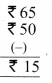Money Suma needs in adition = ₹ 15
So suma cannot buy a pumpkin

Question 3.
If, Suma wants to buy a dozen lemons with ₹ 30. how much money will she get back ?
The amount at money Suma have = ₹ 30
Cost 1 dozen lemons = ₹ 26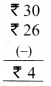The amount she will get back = ₹ 4

Question 4.
What is the difference between the price of 1 kg. of brinjals and one snake gourd?
Cost of 1 Kg brinjal = ₹ 30
Cost of each snake guard = ₹ 18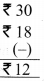Difference between their costs = ₹ 12

Textbook Page No. 9

Example: In the shop, there is a cupboard of 4 rows and 5 columns of shelves. How mans’ shelves are there?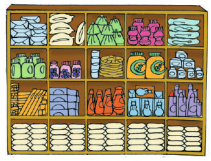Number of shelves = 4 × 5 = 20
There are 4rows in the cupboard. In each row there are 5 shelves. In each shelf there are 10 salt packets. How many salt packets are there In total?
Number of salt packets = 5 × 10= 50

Do These

Do the following:

a)
2 × 1 = ____ ; 3 × 4 = ____ ; 5 × 3 = ____ ; 4 × 6 = _____
2 ; 12 ; 15 ; 24

4 × 8 = ____ ; 4 × 0 = ____ ; 10 × 3 = ____ ; 10 × 5 = _____
32 ; 0 ; 30 ; 50

10 × 9 = ____ ; 5 × 9 = ____ ; 5 × 5 = ____ ; 2 × 8 = _____
90 ; 45 ; 25 ; 16b) Manu give 5 biscuits each of her 4 friends. How man biscuits are there in total?
Number of friends Manu have = 4
Number of biscuits manu gave to each friend = 5
Number of biscuits there in totally
= 4 × 5
= 20 biscuits

c) In a game, Avinash won 5 marbles from each of his 6 friends. How many marbles did Avinash won?
Number of friends Avinash have = 6
Number of marbles
Avinash won from each friend = 5
Number of marbles Avinash won
= 6 × 5 = 30 marbles

d) Manu’s age is 8 years. If we multiply Manu’s age wIth 5, we get her mother’s age. What Is the age of her mother?
Age of Manu = 8 years
Age of manu mother times of Manu = 5
= 5 × 8 = 40 years

Textbook Page No. 10

Do these :

1.  Do the following.

a) 4 ÷ 2 = ____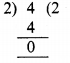b) 6 ÷ 3 = ____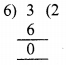c) 15 ÷ 5 = ____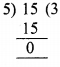d) 18 ÷ 3 = ____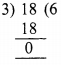e) 24 ÷ 4 = ____
6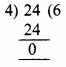f) 35 ÷ 5 = ____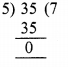g) 49 ÷ 7 = ____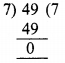h) 72 ÷ 8 = ____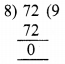Question 2.
The cost of an eraser is ₹ 4. How many erasers can you get for ₹ 20 ?
Total amount = ₹ 20
Cost of each eraser = ₹ 4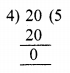Number of erasers I get = 20 ÷ 4
= 5 erasersQuestion 3.
There are 16 students in a classroom. If 4 students sit on each bench, how many benches are needed for the students ?
Number of students in a class room = 16
Number of students sit on each bench = 4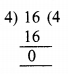Number of benches required = 16 ÷ 4
= 4 benches

Question 4.
In a garden, Sunitha planted 20 salplings. If she planted 5 saplings in each row, how many rows did she plant in ?
Number of sapling planted by Sunitha totally = 20
Number of sapling planted in each row = 5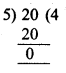Number of rows she planted = 20 ÷ 5 = 4 rows

Question 5.
If the cost of a hair band is ₹ 5, how many hair bands can you get for ₹ 15 ?
Cost of a hair band = ₹ 5
Total Amount = ₹ 20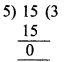Number of hair bands = 20 ÷ 5 = 4 bands

Question 6.
There are 3 chairs in each row. If there are 18 chairs in total, in how many rows were they arranged ?
Total chairs are = 18
Number of chairs in each row = 3
Number of rows were they arranged = 18 ÷ 3 = 6

Textbook Page No. 11

Exercise – 2

1. Write the numbers of the following.
a) Forty six = ____
46

b) Seventy four = ____
74

c) Eight hundred and twenty nine = ____
829

d) Seven hundred and six = ____
706

Question 2.
Write the numbers in words.
a) 37 = _____
Thirty seven

b) 98 = ____
Ninty eight

c) 469 = _____
Four hundred and sixty nine

d) 657 = _______
Six hundred and fifty seven

a)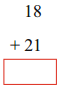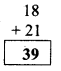b)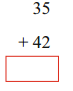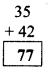c)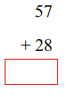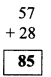d)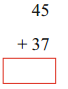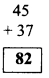e)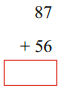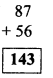4. Do the following Subtractions.

a)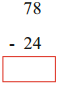b)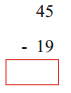c)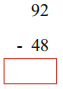d)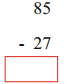e)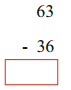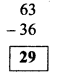5. Do the following multiplications.

a) 2 × 5 = _____
10

b) 3 × 6 = _____
18

c) 5 × 3 = _____
15

d) 4 × 6 = ____
24e) 12 × 2 = ____
24

f) 18 × 5 = ____
90

6. Do the following.

a) 6 ÷ 3 = ____
2

b) 9 ÷ 3 = _____
3

c) 10 ÷ 2 = ____
5

d) 12 ÷ 2 = ____
6

e) 18 ÷ 3 = ____
6

f) 15 ÷ 5 = ____
3

Multiple Choice Questions

Question 1.
Which is the price value of the ‘4’ is in the number 248 ?
A) 4
B) 40
C) 400
D)None
B) 40

Question 2.
Which is the Face value of the ‘3’ is in the number 453 ?
A) 300
B) 30
C) 3
D) None
C) 3Question 3.
Correct ascending order of 77, 12, 26, 33
A) 77, 12, 26, 33
B) 77, 33, 26, 12
C) 12, 26, 33, 77
D) 33,26, 12, 77
C) 12, 26, 33, 77

Quection 4.
Correct descending order of 436, 563, 568, 458
A) 568, 563, 459, 436
B) 436, 459, 563, 568
C) 436, 568, 459, 563
D) 568, 436, 459, 563
A) 568, 563, 459, 436

Quection 5.
Numerical value of “Eight hundred and seventy six”
A) 860
B) 876
C) 867
D) 768